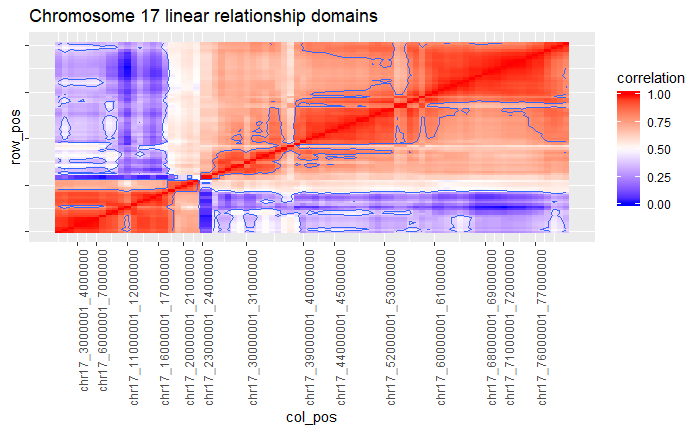This vignette was created to showcase additional cancers and also to highlight additional, less-known features of the package. Additional examples: TCGA-BLCA TARGET-AML TARGET-SKCM TCGA-PRAD

``````if(!dir.exists("extracted_aml_data")){dir.create("extracted_aml_data")}
target_files_aml<-list.files(path = "extracted_aml_data",pattern=glob2rx("*NormalVsPrimary.tsv"),recursive=T,full.names = T)
print(target_files_aml)``````
``````sample_aggregated_segvals_aml<-formSampleMatrixFromRawGDCData(tcga_files = target_files_aml,format = "TARGET")
saveRDS(sample_aggregated_segvals_aml,"aml_sample_matched_input_matrix.rds")``````

Now that we’ve created and saved the AML matrix, let’s visualize it with a quick correlation map of a single chromosome, chromosome 7, the location of the BRAF gene and nearby EZH2 gene. The BRAF gene (chr7:140419127-140624564) is a locus for recurrent copy number aberrations.

Reference: Tarlock et al.; Recurrent Copy Number Variants Are Highly Prevalent in Acute Myeloid Leukemia. Blood 2017; 130 (Supplement 1): 3800.).

Some of the bins are invariant and correlation requires that the standard deviation be a value other than zero (otherwise correlation cannot be calculated). We will remove them in a couple steps and transpose the matrix, making the columns the bins and the samples the rows.

``````sample_aggregated_segvals_aml<-readRDS("aml_sample_matched_input_matrix.rds")
invariant_bins<-which((sample_aggregated_segvals_aml[stringr::str_detect(rownames(sample_aggregated_segvals_aml),"chr7"),] %>% t() %>% as.data.frame() %>% sapply(sd))==0)
chr_7_mat<-sample_aggregated_segvals_aml[(stringr::str_detect(rownames(sample_aggregated_segvals_aml),"chr7") & rownames(sample_aggregated_segvals_aml) %in% setdiff(rownames(sample_aggregated_segvals_aml),names(invariant_bins))),] %>% t()``````

Now we’ll perform correlation on the plot.

``````chr_7_mat %>%  cor(use="pairwise.complete.obs",method="pearson") %>%
CNVScope::signedRescale(max_cap=1) %>%
reshape2::melt()  %>%
ggplot(aes(x=reshape2::colsplit(Var1,"_",c("chr","start","end"))\$start,
y=reshape2::colsplit(Var2,"_",c("chr","start","end"))\$start,
fill=value)) + geom_raster() +
theme(axis.text.x = element_blank(),axis.text.y=element_blank(),axis.title = element_blank()) +
ggplot2::scale_fill_gradient2(low = "blue", high = "red", midpoint = 0.5, limits = c(0, 1))``````
``````## Warning in f(...): Raster pixels are placed at uneven horizontal intervals
## and will be shifted. Consider using geom_tile() instead.``````
``````## Warning in f(...): Raster pixels are placed at uneven vertical intervals
## and will be shifted. Consider using geom_tile() instead.``````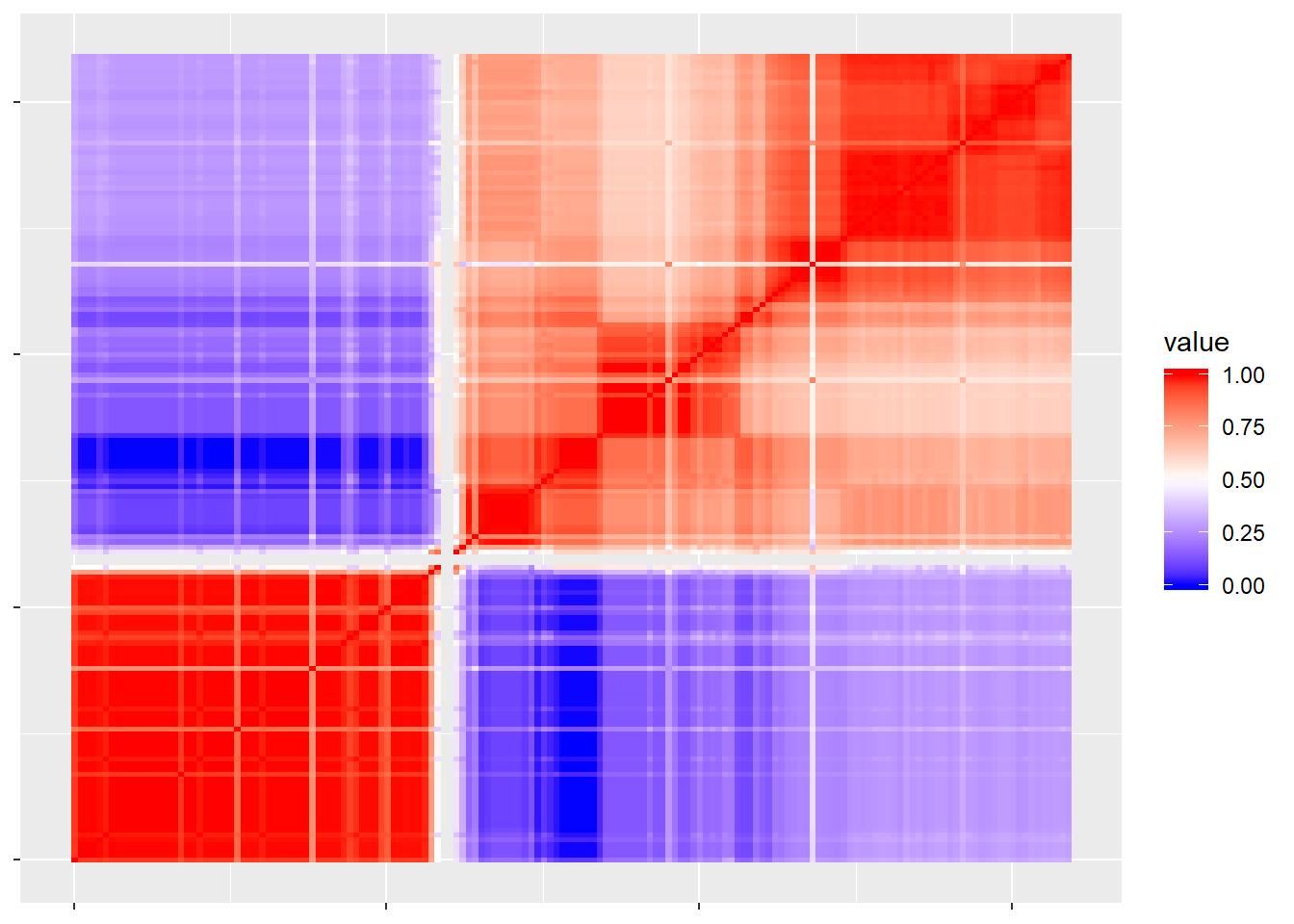We could then utilize our domain finding function to find the borders of domains in the matrix. There’s an obvious distruption near the left center of the matrix (see the blue streaks of anticorrelation?).

``colnames(chr_7_mat)[CNVScope::getAsymmetricBlockIndices(cor(chr_7_mat,use="pairwise.complete.obs"))]``
``````##  "chr7_56000001_57000000"   "chr7_58000001_59000000"
##  "chr7_62000001_63000000"   "chr7_110000001_111000000"
##  "chr7_159000001_159138663"``````
``````breakpoints<-colnames(chr_7_mat)[CNVScope::getAsymmetricBlockIndices(cor(chr_7_mat,use="pairwise.complete.obs"))] %>% stringr::str_split_fixed(string = .,pattern="_",n=3) %>% as.matrix() %>% .[,2] %>% as.numeric()
breakpoint_labels <- colnames(chr_7_mat)[CNVScope::getAsymmetricBlockIndices(cor(chr_7_mat,use="pairwise.complete.obs"))]
breakpoint_labels``````
``````##  "chr7_56000001_57000000"   "chr7_58000001_59000000"
##  "chr7_62000001_63000000"   "chr7_110000001_111000000"
##  "chr7_159000001_159138663"``````

Now, we’ll make another plot, only labeling the breakpoints.

``````chr_7_mat %>%  cor(use="pairwise.complete.obs",method="pearson") %>%
CNVScope::signedRescale(max_cap=1) %>%
reshape2::melt()  %>%
ggplot(aes(x=reshape2::colsplit(Var1,"_",c("chr","start","end"))\$start,
y=reshape2::colsplit(Var2,"_",c("chr","start","end"))\$start,
fill=value)) + geom_raster() +
theme(axis.text.x = element_text(angle=90, hjust=1),axis.text.y=element_blank(),axis.title = element_blank()) +
scale_x_continuous(breaks=breakpoints,labels=breakpoint_labels) +
ggplot2::scale_fill_gradient2(low = "blue", high = "red", midpoint = 0.5, limits = c(0, 1))``````
``````## Warning in f(...): Raster pixels are placed at uneven horizontal intervals
## and will be shifted. Consider using geom_tile() instead.``````
``````## Warning in f(...): Raster pixels are placed at uneven vertical intervals
## and will be shifted. Consider using geom_tile() instead.``````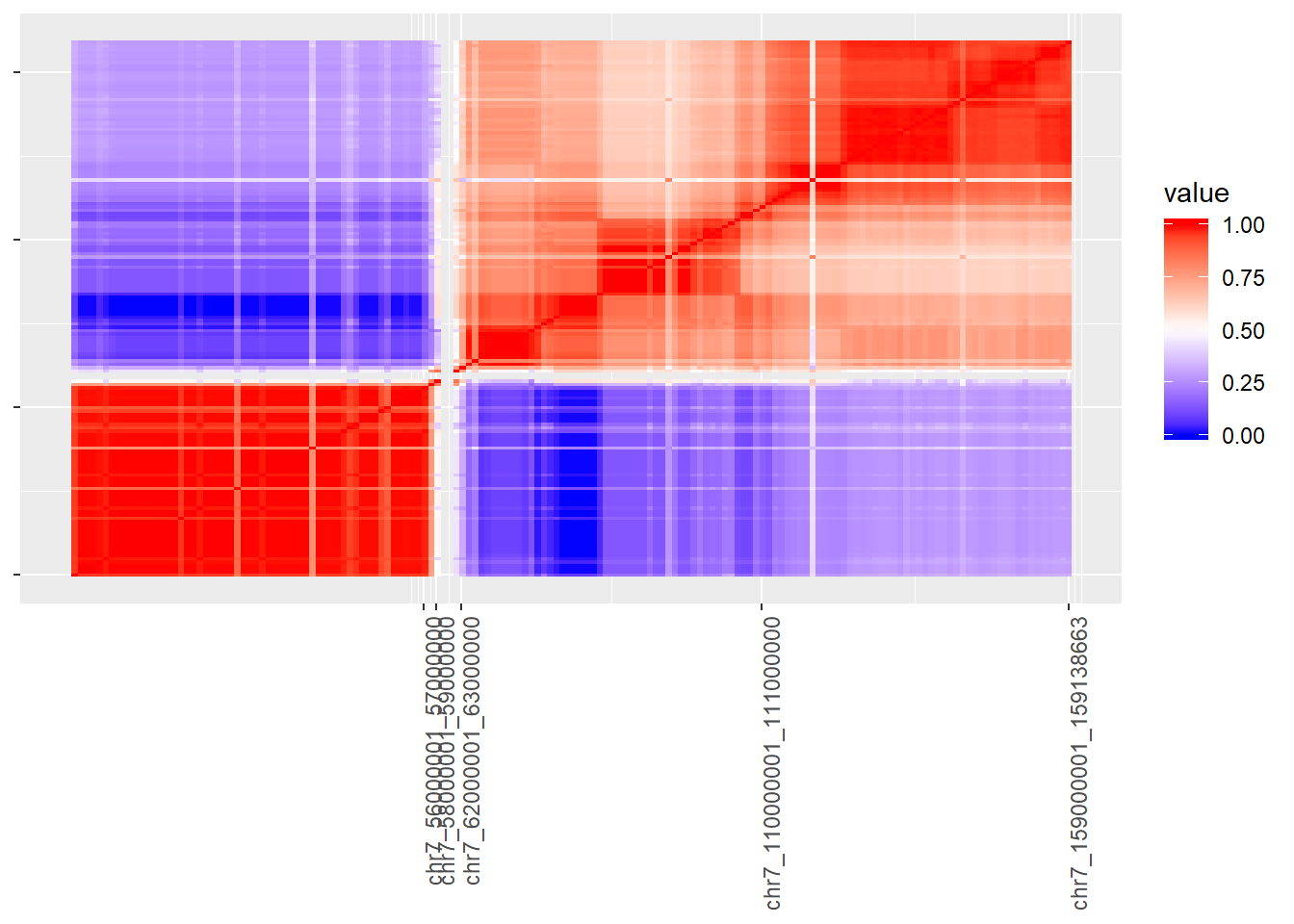Finally, let’s try our probability weighting function for the matrix and see if we can find clearer regions of association. We’ll also try another segmentation algorithm with the jointseg package. In most cases, you can achieve a definite speed increase using the parallel=T option. We have disabled it to build the vignette without warnings.

``````chr_7_probdist <- CNVScope::calcCNVKernelProbDist(cor(chr_7_mat,use="pairwise.complete.obs"),parallel=F)\$percentile_matrix
js_breakpoints<-jointseg::jointSeg(chr_7_probdist,K=20)\$bestBkp
js_breakpoint_labels<-colnames(chr_7_mat)[js_breakpoints]``````

We’ll notice that using this combination of techniques, we’ve managed to pick up an AML driver between domain endpoints, in a large region where the association is less than expected (as determined by the calcCNVprobdist function). Within the region of 115 and 120Mb lies the MET gene, where an LOH was detected in the paper. It’s a pretty obvious signature, perhaps the most obvious in the whole plot.

Further, we suggest that there is an minor signal off the diagonal in the region of this and the location of BRAF (chr7:140419127-140624564, another recurrent CNV in the paper), associated with the border of a region near 16-24Mb.Within this area are the PMS(a tumor suppressor), RAC1 (oncogene), and ETV1 (oncogene). PMS2 alteration has been implicated in AML previously (https://www.ncbi.nlm.nih.gov/pmc/articles/PMC3734905/).

We don’t suggest that this tool is perfect and will rapidly make clear all cancer drivers associated with CNVs, but we suggest that it can, with other tools, add to your investigative toolbox to substantiate known drivers and to elucidate new ones.

``````chr_7_probdist %>%
#  CNVScope::signedRescale(max_cap=1) %>%
reshape2::melt()  %>%
ggplot(aes(x=Var1,
y=Var2,
fill=value)) + geom_tile() +
#  theme(axis.title = element_blank()) + #axis.text.x = element_blank(),axis.text.y=element_blank(),
theme(axis.text.x = element_text(angle=90, hjust=1),
axis.text.y = element_text(angle=0, hjust=1)
,axis.title = element_blank()) +
#    scale_x_continuous(breaks=js_breakpoints,labels=js_breakpoint_labels) +
#      scale_y_continuous(breaks=js_breakpoints,labels=js_breakpoint_labels) +
scale_x_continuous(breaks=js_breakpoints,labels=js_breakpoint_labels) +
scale_y_continuous(breaks=js_breakpoints,labels=js_breakpoint_labels) +

ggplot2::scale_fill_gradient2(low = "blue", high = "red", midpoint = 0.5, limits = c(0, 1))``````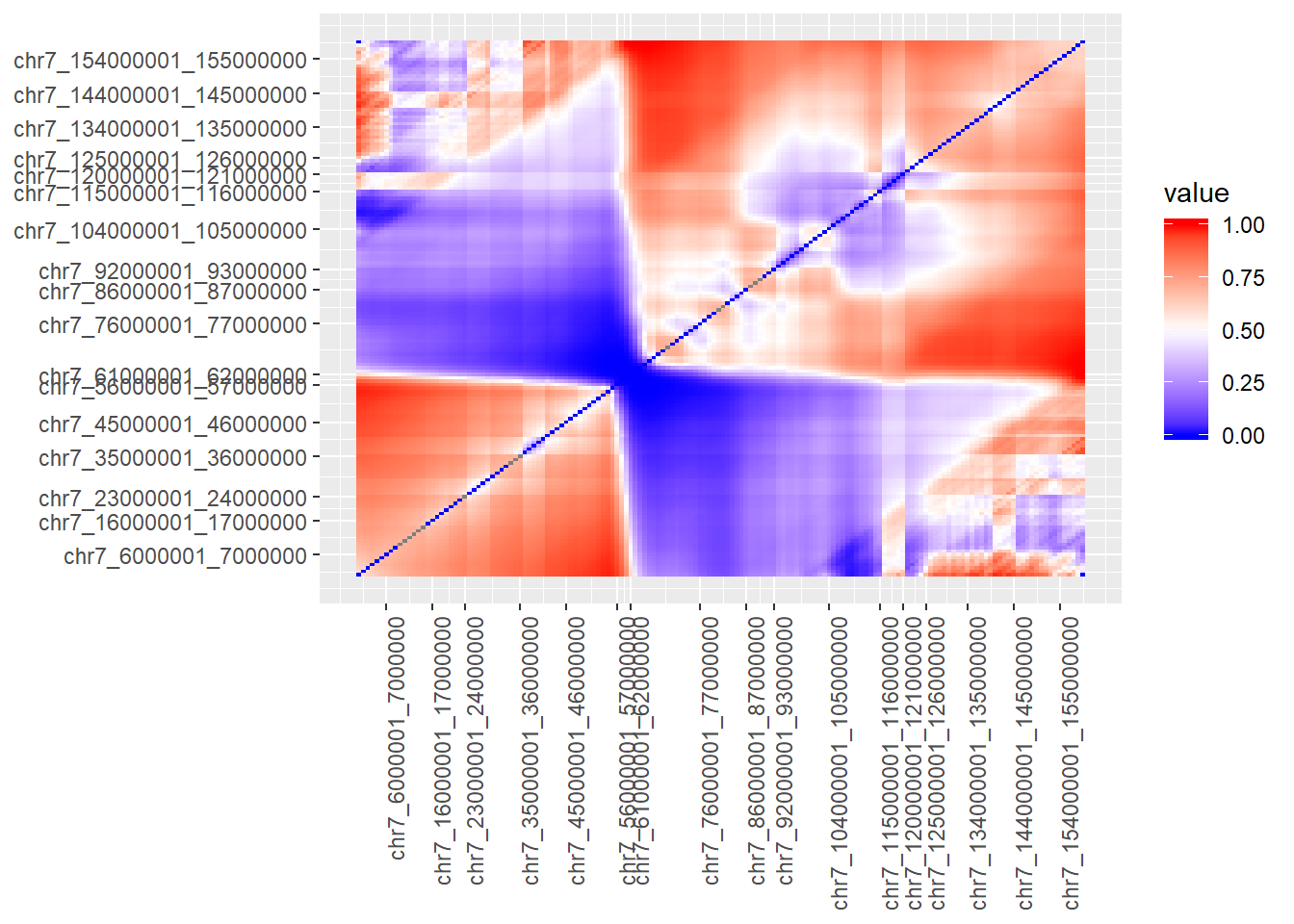Using the below code, we can find a few more genes to explore that are known to be associated with AML in the COSMIC cancer gene census. this requires the CNVScope Public Data package to be installed properly.

``````census_data <- readRDS(system.file("censushg19.rds",package = "CNVScope"))
census_data[census_data@seqnames %in% "chr7"] %>% sort() %>% tibble::as_tibble() %>% janitor::clean_names() %>% dplyr::select(seqnames,start,end,gene_symbol,tumour_types_somatic,tumour_types_germline) %>% dplyr::filter(start>60e6,stringr::str_detect(string = tumour_types_somatic,pattern="AML") | stringr::str_detect(string = tumour_types_germline,pattern="AML"))``````

Now for a TCGA set, let’s try a bladder cancer dataset:

``````if(!dir.exists("extracted_blca_data")){dir.create("extracted_blca_data")
tcga_files_blca<-list.files(path = "extracted_blca_data",pattern=glob2rx("*.tsv"),recursive=T,full.names = T)
print(tcga_files_blca)``````
``````sample_aggregated_segvals_blca<-formSampleMatrixFromRawGDCData(tcga_files = tcga_files_blca,format = "TCGA",parallel=T)
saveRDS(sample_aggregated_segvals_blca,"blca_sample_matched_input_matrix.rds")``````

For bladder cancer (BLCA), we’ll look at ERBB2, mentioned in this article as having amplifications in up to 5% of samples. We may not be able to detect it in our sample, but we can prove that our method did indeed process the TCGA data format.

``````sample_aggregated_segvals_blca<-readRDS("blca_sample_matched_input_matrix.rds")
invariant_bins<-which((sample_aggregated_segvals_blca[stringr::str_detect(rownames(sample_aggregated_segvals_blca),"chr17"),] %>% t() %>% as.data.frame() %>% sapply(sd))==0)
chr_17_mat<-sample_aggregated_segvals_blca[(stringr::str_detect(rownames(sample_aggregated_segvals_blca),"chr17") & rownames(sample_aggregated_segvals_blca) %in% setdiff(rownames(sample_aggregated_segvals_blca),names(invariant_bins))),] %>% t()``````

Now we’ll perform correlation on the plot.

``````chr_17_mat %>%  cor(use="pairwise.complete.obs",method="pearson") %>%
CNVScope::signedRescale(max_cap=1) %>%
reshape2::melt()  %>%
ggplot(aes(x=reshape2::colsplit(Var1,"_",c("chr","start","end"))\$start,
y=reshape2::colsplit(Var2,"_",c("chr","start","end"))\$start,
fill=value)) + geom_raster() +
theme(axis.text.x = element_blank(),axis.text.y=element_blank(),axis.title = element_blank()) +
ggplot2::scale_fill_gradient2(low = "blue", high = "red", midpoint = 0.5, limits = c(0, 1))``````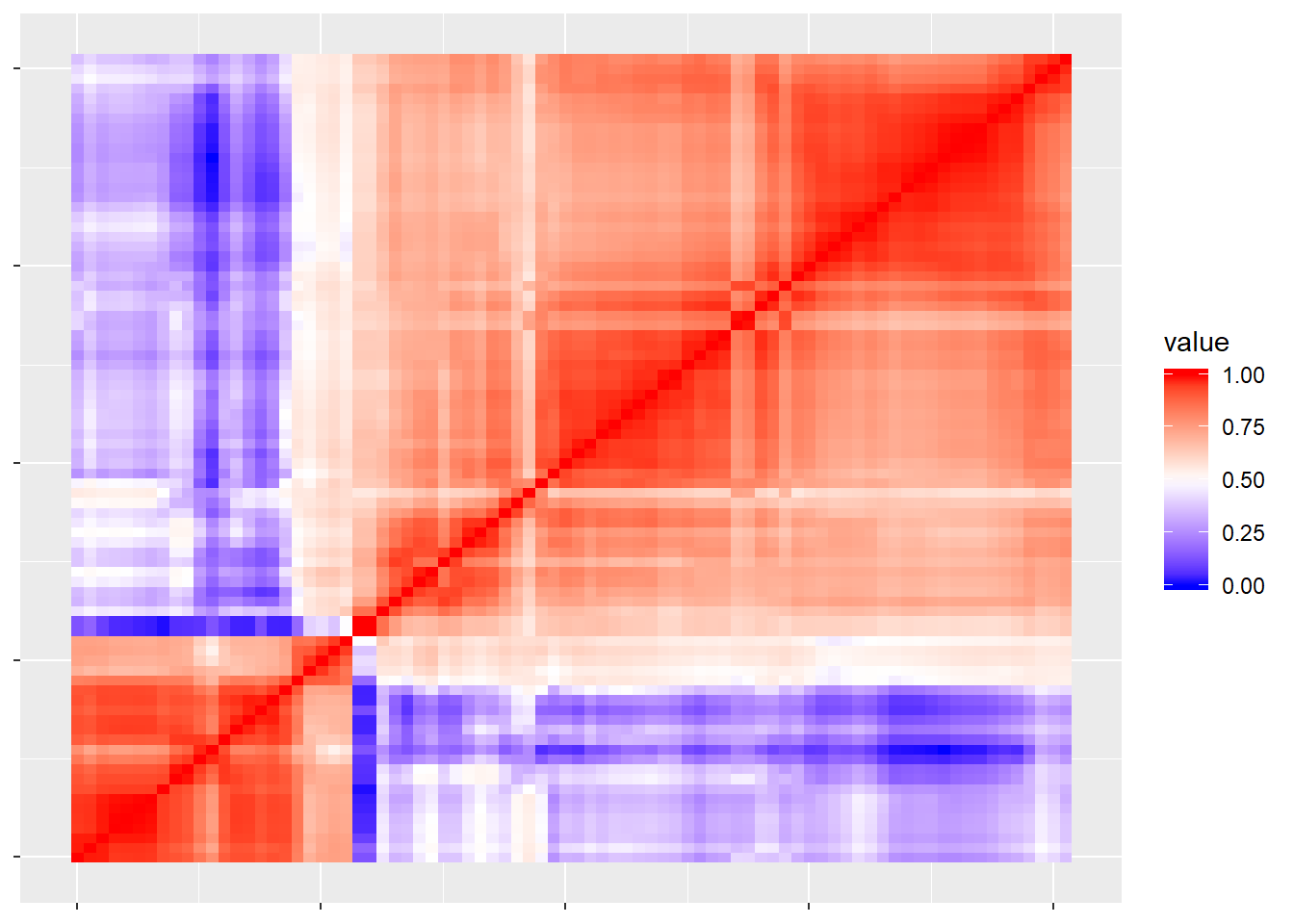We can follow the same procedure with this case, but it may not yield precisely the same dramatic result. We expect with most investigative tools this is the case and do not guarantee a winning result each time. In this case, we are attempting to show that the tool DOES work on TCGA data. We can confidently say that we created a matched sample matrix with the TCGA format as well as the TARGET format. We recommend setting parallel=T on the next line for speed, but for the purposes of compatibility with all systems, we have set the code to run without additional cores in this example.

``````chr_17_probdist <- CNVScope::calcCNVKernelProbDist(cor(chr_17_mat,use="pairwise.complete.obs"),parallel=F)\$percentile_matrix
colnames(chr_17_probdist)<-colnames(chr_17_mat)
rownames(chr_17_probdist)<-colnames(chr_17_mat)
chr_17_js_breakpoints<-jointseg::jointSeg(chr_17_probdist,K=40)\$bestBkp``````
``````## Warning in segmentByRBS(Y, K, ...): No more candidate splits for the
## desired 'minRegionSize': returning with only 33 breakpoints``````
``````chr_17_js_breakpoint_labels<-colnames(cor(chr_17_mat))[chr_17_js_breakpoints]
chr_17_js_breakpoint_labels``````
``````##   "chr17_3000001_4000000"   "chr17_6000001_7000000"
##   "chr17_11000001_12000000" "chr17_16000001_17000000"
##   "chr17_20000001_21000000" "chr17_23000001_24000000"
##   "chr17_30000001_31000000" "chr17_39000001_40000000"
##   "chr17_44000001_45000000" "chr17_52000001_53000000"
##  "chr17_60000001_61000000" "chr17_68000001_69000000"
##  "chr17_71000001_72000000" "chr17_76000001_77000000"``````

With the coordinates for ERBB2 at chr17:37844167-37886679, we find a precise match in the second breakpoint. It lies exactly within it. All the breakpoints are plotted in the plot below. Beyond that, the centromere point is precisely identifiable. It not only shows pathophysiology, but illustrates clearly the position of the chromosomal landmark as the border between the largest domains. Click here to view the ensembl-75 view of this position. It is clear that it precisely matches the location of the centromere. The contours have been added to highlight the domains. We have also plotted the weighted probability of relationships. Finally, we took an approach of investigating correlation differences between spearman (nonlinear) and pearson (linear) correlation. This tends to highlight a specific region in the 37Mb area of the chromosome (near ERBB2).

``````breakpoint_plot_probdist <- chr_17_probdist %>% #  cor(use="pairwise.complete.obs",method="pearson") %>%
CNVScope::signedRescale(max_cap=1) %>%
reshape2::melt()  %>%
dplyr::mutate(col_pos=reshape2::colsplit(Var1,"_",c("chr","start","end"))\$start,
row_pos=reshape2::colsplit(Var2,"_",c("chr","start","end"))\$start,
rel_prob=value) %>%
ggplot(aes(x=col_pos,
y=row_pos,
fill=rel_prob)) + geom_raster() +
theme(axis.text.x = element_text(angle=90, hjust=1),axis.text.y=element_blank()) +
scale_x_continuous(breaks=reshape2::colsplit(chr_17_js_breakpoint_labels,"_",c("chr","start","end"))\$start,labels=chr_17_js_breakpoint_labels) +
ggplot2::scale_fill_gradient2(low = "blue", high = "red", midpoint = 0.5, limits = c(0, 1)) +
labs(x="col_pos",y="row_pos",value="Pearson Correlation:") + ggtitle("Chromosome 17 relationship probability") +
geom_contour(binwidth = .395, aes(z = value))
breakpoint_plot <- chr_17_mat %>%   cor(use="pairwise.complete.obs",method="pearson") %>%
CNVScope::signedRescale(max_cap=1) %>%
reshape2::melt()  %>%
dplyr::mutate(col_pos=reshape2::colsplit(Var1,"_",c("chr","start","end"))\$start,
row_pos=reshape2::colsplit(Var2,"_",c("chr","start","end"))\$start,
correlation=value) %>%
ggplot(aes(x=col_pos,
y=row_pos,
fill=correlation)) + geom_raster() +
theme(axis.text.x = element_text(angle=90, hjust=1),axis.text.y=element_blank()) +
scale_x_continuous(breaks=reshape2::colsplit(chr_17_js_breakpoint_labels,"_",c("chr","start","end"))\$start,labels=chr_17_js_breakpoint_labels) +
ggplot2::scale_fill_gradient2(low = "blue", high = "red", midpoint = 0.5, limits = c(0, 1)) +
labs(x="col_pos",y="row_pos",value="Pearson Correlation:") + ggtitle("Chromosome 17 linear relationship domains") +
geom_contour(binwidth = .395, aes(z = value))
breakpoint_plot_corr_diff <- ((chr_17_mat %>%   cor(use="pairwise.complete.obs",method="spearman"))-(chr_17_mat %>%   cor(use="pairwise.complete.obs",method="pearson"))) %>%
CNVScope::signedRescale(max_cap=1) %>%
reshape2::melt()  %>%
dplyr::mutate(col_pos=reshape2::colsplit(Var1,"_",c("chr","start","end"))\$start,
row_pos=reshape2::colsplit(Var2,"_",c("chr","start","end"))\$start,
corr_diff=value) %>%
ggplot(aes(x=col_pos,
y=row_pos,
fill=corr_diff)) + geom_raster() +
theme(axis.text.x = element_text(angle=90, hjust=1),axis.text.y=element_blank()) +
scale_x_continuous(breaks=reshape2::colsplit(chr_17_js_breakpoint_labels,"_",c("chr","start","end"))\$start,labels=chr_17_js_breakpoint_labels) +
ggplot2::scale_fill_gradient2(low = "blue", high = "red", midpoint = 0.5, limits = c(0, 1)) +
labs(x="col_pos",y="row_pos",value="Pearson Correlation:") + ggtitle("Chromosome 17 nonlinear (red) relationship regions, inferred by nonlinear-linear correlation difference") +
geom_contour(binwidth = .395, aes(z = value))

breakpoint_plot
breakpoint_plot_probdist
breakpoint_plot_corr_diff``````